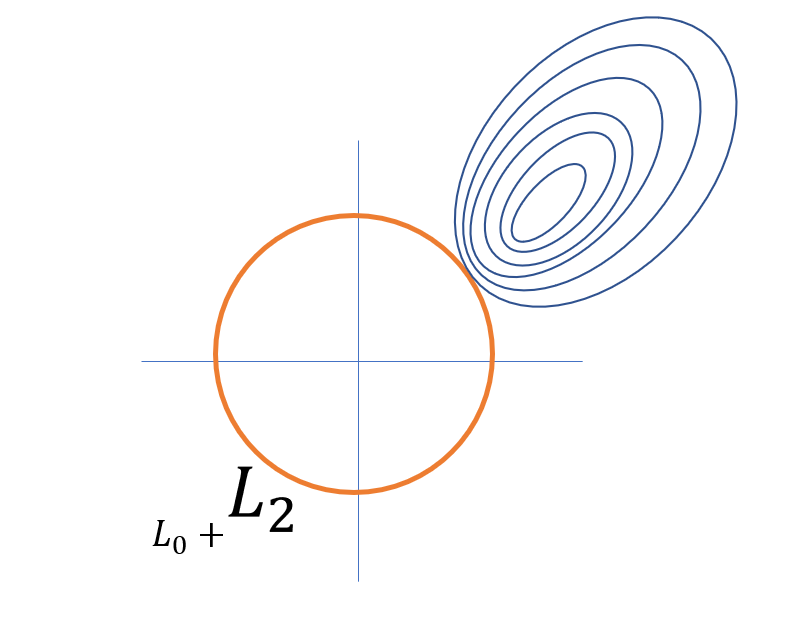# Regularized Best Subset Selection¶In some cases, especially under low signal-to-noise ratio (SNR) setting or predictors are highly correlated, the vallina type of $$\ell_0$$ constrained model may not be satisfying and a more sophisticated trade-off between bias and variance is needed. Under this concern, the abess package provides option of best subset selection with $$\ell_2$$ norm regularization called the regularized best-subset selection (RBESS). The model has this following form:

$\arg\min_\beta L(\beta) + \alpha \|\beta\|_2^2,\; \textup{s.t.}\ ||\beta||_{0}\leq s.$

To implement the RBESS, user need to specify a value to an additive argument alpha in the LinearRegression() function (or other methods). This value corresponds to the penalization parameter in the model above.

Let’s test the RBESS against the no-regularized one over 100 replicas in terms of prediction performance. With argument snr in make_glm_data(), we can add white noise into generated data.

import numpy as np
from abess.datasets import make_glm_data
from abess.linear import LinearRegression
from sklearn.model_selection import train_test_split

np.random.seed(0)

loss = np.zeros((2, 100))
coef = np.repeat([1, 0], [5, 25])
for i in range(100):
np.random.seed(i)
data = make_glm_data(n=100, p=30, k=5, family='gaussian', coef_=coef, snr=0.5, rho=0.5)
train_x, test_x, train_y, test_y = train_test_split(
data.x, data.y, test_size=0.5, random_state=i)

# normal
model = LinearRegression()
model.fit(train_x, train_y)
loss[0, i] = np.linalg.norm(model.predict(test_x) - test_y)
# regularized
model = LinearRegression(alpha=0.1)
model.fit(train_x, train_y)
loss[1, i] = np.linalg.norm(model.predict(test_x) - test_y)

print("The average predition error under best-subset selection:", np.mean(loss[0, :]))
print("The average predition error under regularized best-subset selection:", np.mean(loss[1, :]))

The average predition error under best-subset selection: 32.053166506274785
The average predition error under regularized best-subset selection: 32.08635372450171


We see that the regularized best subset select ("RABESS") indeed reduces the prediction error.

The abess R package also supports regularized best-subset selection. For R tutorial, please view https://abess-team.github.io/abess/articles/v07-advancedFeatures.html.

sphinx_gallery_thumbnail_path = 'Tutorial/figure/regularized_cover.png'

Total running time of the script: ( 0 minutes 0.362 seconds)

Gallery generated by Sphinx-Gallery